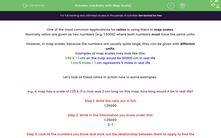# Use Ratio with Map Scales

In this worksheet, students will apply map scales to find the scale values of real life elements or the real values of scaled elements on maps.Key stage:  KS 4

Year:  GCSE

GCSE Subjects:   Maths

GCSE Boards:   AQA, Eduqas, Pearson Edexcel, OCR,

Curriculum topic:   Ratio, Proportion and Rates of Change, Mensuration

Curriculum subtopic:   Ratio, Proportion and Rates of Change Units and Measurement

Difficulty level:#### Worksheet Overview

One of the most common applications for ratios is using them in map scales.

Normally ratios are given as two numbers (e.g. 1:5000) where both numbers must have the same units.

However, in map scales, because the numbers are usually quite large, they can be given with different units.

Examples of map scales may look like this:

1:50 k > 1 cm on the map would be 50000 cm in real life

1 cm:5 miles > 1 cm represents 5 miles in real life

Let's look at these ratios in action now in some examples.

e.g. A map has a scale of 1:25 k. If a river was 2 cm long on this map, how long would it be in real life?

Step 1: Write the ratio out in full:

1:25000

Step 2: Write in the information you know under this:

1:25000

2: ?

Step 3: Look at the numbers you know and work out the relationship between them to apply to find the missing number:

In this case, we multiply 1 by 2 to reach 2 so our rule is '× 2'.

1:25000 × 2

2:50000

This means the river is 50,000 cm long in real life.

e.g. Two car parks are 15 miles apart. If the scale for a map is 1 cm: 5 miles, how far apart are the car parks on the map?

Step 1: Write the ratio out in full:

1 cm:5 miles

Step 2: Write in the information you know under this:

1 cm:5 miles

?:15 miles

Step 3: Look at the numbers you know and work out the relationship between them to apply to find the missing number:

5 × 15 = 3 so our rule here is '× 3'.

1 cm:5 miles

3 cm:15 miles

This means the car parks are 3 cm apart on the map.

In this activity, we will apply map scales to find the scale values of real life elements or the real values of scaled elements on maps.

### What is EdPlace?

We're your National Curriculum aligned online education content provider helping each child succeed in English, maths and science from year 1 to GCSE. With an EdPlace account you’ll be able to track and measure progress, helping each child achieve their best. We build confidence and attainment by personalising each child’s learning at a level that suits them.

Get started